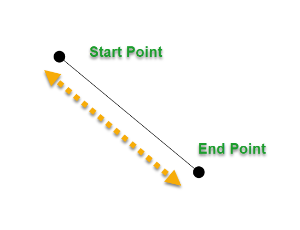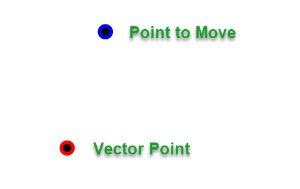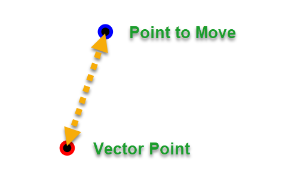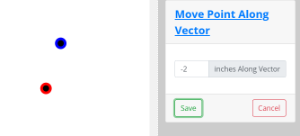Vector is a term that you will find throughout the Fashioner program. We are most concerned with direction, when we use this term.

## Two Point Vectors

Moving a point horizontally or vertically is easy. You simply move in the X or Y axis a given value. However, there are times when you need to move a point and keep it's current orientation in regard to another point. Let's look at an example.Here is a line with a start point and end point. This line is 20 units long.

If we want to make it 5 units longer by moving the start point. We have to move the start point following the direction of the line. That direction is the vector and it is indicated by the dashed yellow line.

There are many tools that refer to vectors, here is one called Move Point Along Vector. This tool allows you to select two points and move one using the other as a vector point.In this case, there is no line, just the two points. We can draw an imaginary line to see the vector.Here is the same two points. The point to move has been moved -2 inches along the vector.Don't be confused by fancy terms. Vector just means direction and generally refers to items that are not in a straight line vertically or horizontally.

## Curve Vectors

Calculating the vector of a point on a curve is trickier. The Fashioner will move a very tiny distance back along the curve from the selected point and create a virtual point. Then it will calculate the vector using those two points.